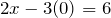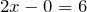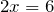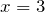# Graphing a Linear Equation

Equations whose graphs are straight lines are called linear equations. The following are some examples of linear equations:

2x − 3y = 6   ,   3x = 4y − 7   ,   y = 2x − 5   ,   2y = 3   ,    and   x − 2 = 0.

A line is completely determined by two points. Therefore, to graph a linear equation, we need to find the coordinates of two points. This can be accomplished by choosing an arbitrary value for x or y and then solving for the other variable.

Example 2.1.1

Graph the line: y = 3x + 2

Solution

We need to find the coordinates of at least two points.

We arbitrarily choose x = -1 , x = 0 , and x = 1.

If x = -1, then y = 3(-1) + 2 or -1. Therefore, (-1, -1) is a point on this line.

If x = 0, then y = 3(0) + 2 or y = 2. Hence the point (0, 2).

If x = 1, then y = 5, and we get the point (1, 5). Below, the results are summarized, and the line is graphed.

 X -1 0 1 Y -1 2 5Example 2.1.2

Graph the line: 2x + y = 4
Solution
Again, we need to find coordinates of at least two points.
We arbitrarily choose x = -1, x = 0 and y = 2.
If x = -1, then 2(-1) + y = 4 which results in y = 6. Therefore, (-1, 6) is a point on this line.
If x = 0, then 2(0) + y = 4, which results in y = 4. Hence the point (0, 4).

If y = 2, then 2x + 2 = 4, which yields x = 1, and gives the point (1, 2). The table below shows the points, and the line is graphed.

 x -1 0 1 y 6 4 2The points at which a line crosses the coordinate axes are called the intercepts. When graphing a line, intercepts are preferred because they are easy to find. In order to find the x-intercept, we let y = 0, and to find the y-intercept, we let x = 0.

Example 2.1.3

Find the intercepts of the line: 2x − 3y = 6, and graph.
Solution
To find the x-intercept, we let y = 0 in our equation, and solve for x.Therefore, the x-intercept is 3.
Similarly by letting x = 0, we obtain the y-intercept which is -2.
Note: If the x-intercept is 3, and the y-intercept is -2, then the corresponding points are (3, 0) and (0, -2), respectively.In higher math, equations of lines are sometimes written in parametric form. For example, x = 3 + 2t , y = 1 + t. The letter t is called the parametre or the dummy variable. Parametric lines can be graphed by finding values for x and y by substituting numerical values for t.

Example 2.1.4

Graph the line given by the parametric equations: x = 3 + 2t , y = 1 + t
Solution
Let t = 0, 1 and 2, and then for each value of t find the corresponding values for x and y.

The results are given in the table below.

 t 0 1 2 x 3 5 7 y 1 2 3# Horizontal and Vertical Lines

When an equation of a line has only one variable, the resulting graph is a horizontal or a vertical line.

The graph of the line x = a, where a is a constant, is a vertical line that passes through the point ( a, 0). Every point on this line has the x-coordinate a, regardless of the y-coordinate.

The graph of the line y = b, where b is a constant, is a horizontal line that passes through the point (0, b). Every point on this line has the y-coordinate b, regardless of the x-coordinate.

Example 2.1.5

Graph the lines: x = -2 , and y = 3.
Solution
The graph of the line x = -2 is a vertical line that has the x-coordinate -2 no matter what the y-coordinate is. Therefore, the graph is a vertical line passing through (-2, 0).
The graph of the line y = 3, is a horizontal line that has the y-coordinate 3 regardless of what the x-coordinate is. Therefore, the graph is a horizontal line that passes through (0, 3).Note: Most students feel that the coordinates of points must always be integers. This is not true, and in real life situations, not always possible. Do not be intimidated if your points include numbers that are fractions or decimals.

# Practice questions

1. Is the point (2, 3) on the line 5x – 2y = 4?

2. For the line 3xy = 12, complete the following ordered pairs:

a. (2, ?) (? , 6)

b. (0, ?) (?, 0)

3. Graph y = 4x – 3

4. Graph 2x + 4 = 0

5. Graph the line using the parametric equations: x = 1 + 2t; y = 3 + t.

6. Graph the following three equations on the same set of coordinate axes: y = x + 1; = 2x + 1; y = –+ 1.